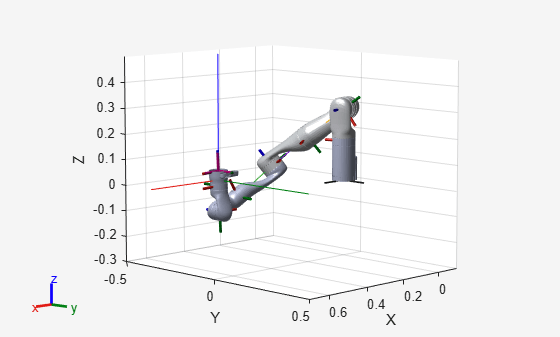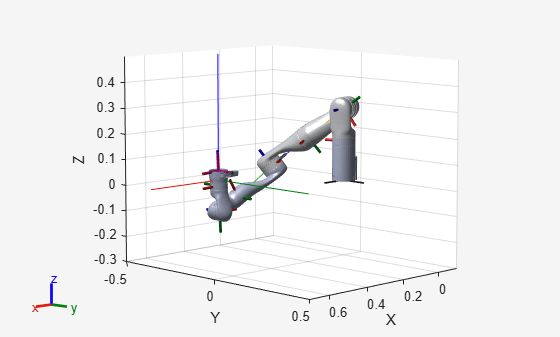# Generate Code for Inverse Kinematics Computation Using Robot from Robot Library

This example shows how to perform code generation to compute Inverse Kinematics (IK) using robots from the robot library. For this example, you can use an `inverseKinematics` object with an included `rigidBodyTree` robot model using `loadrobot` to solve for robot configurations that achieve a desired end-effector position.

A circular trajectory is created in a 2-D plane and given as points to the generated MEX inverse kinematics solver. The solver computes the required joint positions to achieve this trajectory. Finally, the robot is animated to show the robot configurations that achieve the circular trajectory.

### Write Algorithm to Solve Inverse Kinematics

Create a function, `ikCodegen`, that runs the inverse kinematics algorithm for a KINOVA® Gen3 robot model created using `loadrobot`.

```function qConfig = ikCodegen(endEffectorName,tform,weights,initialGuess) %#codegen robot = loadrobot("kinovaGen3","DataFormat","row"); ik = inverseKinematics('RigidBodyTree',robot); [qConfig,~] = ik(endEffectorName,tform,weights,initialGuess); end ```

The algorithm acts as a wrapper for a standard inverse kinematics call. It accepts standard inputs, and returns a robot configuration solution vector. Since you cannot use a handle object as the input or output to a function that is supported for code generation. Load the robot inside the function. Save the `ikCodegen` function in your current folder.

### Verify Inverse Kinematics Algorithm in MATLAB

Verify the IK algorithm in MATLAB before generating code.

Load a predefined KINOVA® Gen3 robot model as r`igidBodyTree` object. Set the data format to `"row"`.

`robot = loadrobot("kinovaGen3","DataFormat","row");`

Show details of the robot.

`showdetails(robot)`
```-------------------- Robot: (8 bodies) Idx Body Name Joint Name Joint Type Parent Name(Idx) Children Name(s) --- --------- ---------- ---------- ---------------- ---------------- 1 Shoulder_Link Actuator1 revolute base_link(0) HalfArm1_Link(2) 2 HalfArm1_Link Actuator2 revolute Shoulder_Link(1) HalfArm2_Link(3) 3 HalfArm2_Link Actuator3 revolute HalfArm1_Link(2) ForeArm_Link(4) 4 ForeArm_Link Actuator4 revolute HalfArm2_Link(3) Wrist1_Link(5) 5 Wrist1_Link Actuator5 revolute ForeArm_Link(4) Wrist2_Link(6) 6 Wrist2_Link Actuator6 revolute Wrist1_Link(5) Bracelet_Link(7) 7 Bracelet_Link Actuator7 revolute Wrist2_Link(6) EndEffector_Link(8) 8 EndEffector_Link Endeffector fixed Bracelet_Link(7) -------------------- ```

Specify the end-effector name, the weights for the end-effector transformation, and the initial joint positions.

```endEffectorName = 'EndEffector_Link'; weights = [0.25 0.25 0.25 1 1 1]; initialGuess = [0 0 0 0 0 0 0];```

Call the inverse kinematics solver function for the specified end-effector transformation.

```targetPose = trvec2tform([0.35 -0.35 0]); qConfig = ikCodegen(endEffectorName,targetPose,weights,initialGuess)```
```qConfig = 1×7 1.3085 2.2000 -1.3011 1.0072 -1.1144 2.0500 -3.2313 ```

Visualize the robot with the computed robot configuration solution.

```figure; show(robot,qConfig); hold all plotTransforms(tform2trvec(targetPose),tform2quat(targetPose),"FrameSize",0.5);```### Generate Code for Inverse Kinematics Algorithm

You can use either the `codegen` (MATLAB Coder) function or the MATLAB Coder (MATLAB Coder) app to generate code. For this example, generate a MEX file by calling `codegen` at the MATLAB command line. Specify sample input arguments for each input to the function using the `-args` input argument.

Call the `codegen` function and specify the input arguments in a cell array. This function creates a separate `ikCodegen_mex` function to use. You can also produce C code by using the options input argument. This step can take some time.

`codegen ikCodegen -args {endEffectorName,targetPose,weights,initialGuess}`
```Code generation successful. ```

### Verify Results Using Generated MEX Function

Call the MEX version of the IK solver for the specified transform.

```targetPose = trvec2tform([0.35 -0.35 0]); qConfig = ikCodegen_mex(endEffectorName,targetPose,weights,initialGuess)```
```qConfig = 1×7 1.3085 2.2000 -1.3011 1.0072 -1.1144 2.0500 -3.2313 ```

Visualize the robot with the robot configuration computed using the MEX version of the IK solver.

```figure; show(robot,qConfig); hold all plotTransforms(tform2trvec(targetPose),tform2quat(targetPose),"FrameSize",0.5);```### Compute Inverse Kinematics with MEX function

Use the generated MEX function to compute the Inverse Kinematics solution to achieve a trajectory.

#### Define Trajectory

Create a circular trajectory.

```t = (0:0.2:10)'; % Time count = length(t); center = [0.3 0.1 0]; radius = 0.15; theta = t*(2*pi/t(end)); points = center + radius*[cos(theta) sin(theta) zeros(size(theta))];```

#### Inverse Kinematics Solution

Preallocate configuration solutions as a matrix `qs`. Specify the weights for the end-effector transformation and the end-effector name.

```q0 = [0 0 0 0 0 0 0]; ndof = length(q0); qs = zeros(count,ndof); weights = [0 0 0 1 1 1]; endEffector = 'EndEffector_Link';```

Loop through the trajectory of points to trace the circle. Use the `ikCodegen_mex` function to calculate the solution for each point to generate the joint configuration that achieves the end-effector position. Store the configurations for later use.

```qInitial = q0; % Use home configuration as the initial guess for i = 1:count % Solve for the configuration satisfying the desired end-effector % position point = points(i,:); qSol = ikCodegen_mex(endEffector,trvec2tform(point),weights,qInitial); % Store the configuration qs(i,:) = qSol; % Start from prior solution qInitial = qSol; end```

#### Animate Solution

Once you generate all the solutions, animate the results. You must recreate the robot because it was originally defined inside the function. Iterate through all the solutions. Set the `"FastUpdate"` option of the `show` method to `true` to get a smooth animation.

```robot = loadrobot("kinovaGen3","DataFormat","row"); % Show first solution and set view. figure show(robot,qs(1,:)); view(3) ax = gca; ax.Projection = 'orthographic'; hold on plot(points(:,1),points(:,2),'k') axis([-0.1 0.7 -0.3 0.5]) % Iterate through the solutions framesPerSecond = 15; r = rateControl(framesPerSecond); for i = 1:count show(robot,qs(i,:),'PreservePlot',false,"FastUpdate",true); drawnow waitfor(r); end```